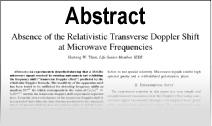Enter the content which will be displayed in sticky bar

# AbstractQuantumlike Chaos in Prime Number Distribution and in Turbulent Fluid Flows

Year: 2001 Pages: 36
Keywords: quantum-like chaos in prime numbers, fractal structure of primes, quantification of prime number distribution, prime numbers and fluid flows
Recent studies by mathematicians and physicists have identified a close association between the distribution of prime numbers and quantum mechanical laws governing the subatomic dynamics of quantum systems such as the electron or the photon. It is now recognised that Cantorian fractal space-time fluctuations characterise dynamical systems of all space-time scales ranging from the microscopic subatomic dynamics to macro-scale turbulent fluid flows such as atmospheric flows. The spacing intervals of adjacent prime numbers also exhibit fractal (irregular) fluctuations generic to dynamical systems in nature. The apparently irregular (chaotic) fractal fluctuations of dynamical systems, however, exhibit self-similar geometrical pattern and are associated with inverse power-law form for the power spectrum. Self-similar fluctuations imply long-range space-time correlations identified as self-organized criticality. A cell dynamical system model for atmospheric flows developed by the author gives the following important results: (a) Self-organized criticality is a signature of quantum-like chaos (b) The observed self-organized criticality is quantified in terms of the universal inverse power-law form of the statistical normal distribution (c) The spectrum of fractal fluctuations is a broadband continuum with embedded dominant eddies. The cell dynamical system model is a general systems theory applicable to all dynamical systems (real world and computed) and the model concepts are applied to derive the following results for the observed association between prime number distribution and quantum-like chaos. (i) Number theoretical concepts are intrinsically related to the quantitative description of dynamical systems. (ii) Continuous periodogram analyses of different sets of adjacent prime number spacing intervals show that the power spectra follow the model predicted universal inverse power-law form of the statistical normal distribution. The prime number distribution therefore exhibits self-organized criticality, which is a signature of quantum-like chaos. (iii) The continuum real number field contains unique structures, namely, prime numbers, which are analogous to the dominant eddies in the eddy continuum in turbulent fluid flows.